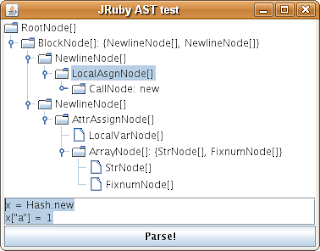## Saturday, November 10, 2007

### Exploring JRuby Syntax Trees With Scala, Part 2

In this post I'm going to show a couple of examples of using Scala to extract elements of Ruby programs.

Because of its functional influence Scala provides nice features to manipulate tree structures. As I mentioned in previous posts, I think one of the most interesting features provided by Scala is pattern matching. Among other pattern matching constructs, Scala provides one that I particularly like : Extractors.

The Matching Object With Patterns paper by Burak Emir , Martin Odersky , and John Williams, describes several techniques for exploring complex object data structures. One of this techniques is the Visitor design pattern which is one of the most used techniques that is used to navigate a syntax tree structure. I think the pattern matching approach can be combined with the visitors to solve different tasks.

In this post I'm interested in showing pattern matching using Scala extractors on the JRuby AST. The JRuby AST seems to provide nice visitor mechanism which will be explored in future posts.

Scala extractor objects allow existing Scala or Java Platform classes to participate in pattern matching just as case classes. Extractor objects can be used to expose only interesting elements for a certain problem and also makes the definition of the class independent of the way it is used in a `match` construct. A nice description of this feature for Scala is provided in the Matching Object With Patterns paper. A similar feature is implemented in F# as active patterns.This concept was originated by work on Views by Philip Walder .

I'm going to show a couple of examples of using this feature with the JRuby AST.

Example 1:

Say that you want to identify the key value that is given to Hash assignment: (here highlighted in red)

``x["a"] = 1``

We need to define extractors for related objects. In order to do this I'm going to use the tool from the previous post to see which classes we need to represent.By reading this code we noticed that we need at least extractors for

• BlockNode

• NewlineNode

• AttrAssignNode

• LocalVarNode

• ArrayNode

• StrNode

• FixnumNode

Basic extractors for the child nodes of these elements could be defined as follows:

``object NewlineNode {  def unapply(astNode : Node) =    if (astNode.isInstanceOf[NewlineNode]) {            Some(astNode.asInstanceOf[NewlineNode].getNextNode)    } else {      None    }}object LocalVarNode {  def unapply(astNode : Node) = {    if (astNode.isInstanceOf[LocalVarNode])  {      Some (astNode.asInstanceOf[LocalVarNode].getName)    } else {      None    }  }} object StrNode {  def unapply(astNode : Node) = {    if (astNode.isInstanceOf[StrNode]) {      Some (astNode.asInstanceOf[StrNode].getValue)    } else {      None    }  }}object BlockNode {  def unapply(astNode : Node) = {     if (astNode.isInstanceOf[BlockNode]) {                     Some (List.fromIterator(               new JavaIteratorWrapper[Node](                     astNode.asInstanceOf[BlockNode].childNodes.iterator())))     } else {       None     }  }}object FixnumNode {  def unapply(astNode : Node) = {    if (astNode.isInstanceOf[FixnumNode]) {      Some (astNode.asInstanceOf[FixnumNode].getValue)    } else {      None    }  }}object AttrAssignNode {  def unapply(astNode : Node) = {    if (astNode.isInstanceOf[AttrAssignNode]) {      val attAsgn = astNode.asInstanceOf[AttrAssignNode]      Some (attAsgn.getReceiverNode,attAsgn.getArgsNode)    } else {      None    }  }}object ArrayNode {  def unapply(astNode : Node) =    if (astNode.isInstanceOf[ArrayNode]) {      val theArrayNode = astNode.asInstanceOf[ArrayNode]      Some(List.fromIterator(new JavaIteratorWrapper[Node](theArrayNode.childNodes.iterator)))    } else {      None    }}``

By defining these extractors we can combine them with existing Scala pattern matching constructs to create the required pattern:

``val rubyCode2 = "x = Hash.new\n"+"x[\"a\"] = 1"    val rootNode2 = r.parse(rubyCode2,"dummy.rb",r.getCurrentContext.getCurrentScope,0).asInstanceOf[RootNode]rootNode2.getBodyNode match {  case BlockNode(           List(_,                NewlineNode(                     AttrAssignNode(                         LocalVarNode("x"),                         ArrayNode(                             List(key,                                  FixnumNode(1))))))) =>     System.out.println("Matches for: "+ key.toString())  case _ => System.out.println("No Match!")}``

Example 2:

For the next example say that we want to extract the identifier which is compared to some number in the condition of an `if` statement. For example:

``if the_variable == 1 then  ...else  ...end``

The tree representation of a code similar to this looks like:The following extractors will be used to identify this pattern:

``object IfStatement {  def unapply(astNode : Node) =    if (astNode.isInstanceOf[IfNode]) {      val theIfNode = astNode.asInstanceOf[IfNode]      Some (theIfNode.getCondition,theIfNode.getThenBody,theIfNode.getElseBody)    } else {      None    }}object EqualComparison {   def unapply(astNode : Node) =     if (astNode.isInstanceOf[CallNode]) {       val theCallNode = astNode.asInstanceOf[CallNode]       if (theCallNode.getName.equals("==") &&           theCallNode.getArgsNode.isInstanceOf[ArrayNode] &&           theCallNode.getArgsNode.asInstanceOf[ArrayNode].size == 1) {           Some (theCallNode.getReceiverNode,                 theCallNode.getArgsNode.asInstanceOf[ArrayNode].get(0))       } else {           None       }     } else {       None     }}``

Note that in this case I'm using a shortcut, instead of defining an extractor for `CallNode` and using it with `ArrayNode` a single extractor is used to identify the binary equal comparison.

By using this extractors we can define the pattern as follows:

``val rubyCode3 = "if the_variable == 30 then\n puts 2\nelse\n puts 3\nend"    val rootNode3 = r.parse(rubyCode3,"dummy.rb",r.getCurrentContext.getCurrentScope,0).asInstanceOf[RootNode]val nodeToMatch = rootNode3.getBodyNode.asInstanceOf[NewlineNode].getNextNodenodeToMatch match {    case IfStatement(EqualComparison(leftSide,_ : FixnumNode),                     _,                     _) =>       System.out.println("Matches for: "+ leftSide.toString())    case _ => System.out.println("No Match!")}``

For future post I'm going to be using these extractors to create more examples in other contexts.